# MCAT Physical : Magnetic Fields

## Example Questions

### Example Question #50 : Ap Physics 2

Which of the following influences the emf produced in a wire loop that is rotating in a magnetic field?

More than one of the other options is correct

The material of which the loop is made

The resistance of the loop

The size of the loop

The shape of the loop

The size of the loop

Explanation:

Recall that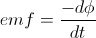, where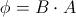. The shape, material characteristics, and resistance do not appear in this equation. So only the size (area) of the loop influences the emf.

### Example Question #533 : Mcat Physical Sciences

Which of the following best describes magnetic field lines?

They always begin at a north pole and terminate at a south pole

More than one of the other options is correct

They show both the relative strength and direction of the magnetic field

They show the direction in which a positive charge will experience a force

They can only cross when multiple magnets are present

They show both the relative strength and direction of the magnetic field

Explanation:

First, magnetic field lines, like electric field lines, can never cross. Also, unlike electric field lines, magnetic field lines are continuous—they do not have starting or ending points. Next, the force experienced by a charge in a magnetic field depends on the charge's velocity direction, not just the magnetic field. So the only remaining choice is that magnetic field lines show both the relative strength and direction of the field.

### Example Question #1 : Magnetism And Electromagnetism

A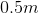current-carrying wire is placed in a uniform magnetic field oriented perpendicularly to the wire. The length of the wire is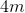and the magnitude of the magnetic field is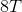. What is the force on the wire?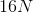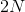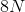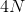Explanation:

We use the formula for force on a wire in a magnetic field: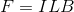Where= force,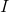= current,= length of wire, and= magnetic mield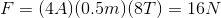### Example Question #535 : Mcat Physical Sciences

A scientist builds a particle accelerator. Which of the following would not help the scientist increase the speed of a particle in the accelerator?

Increasing the charge of the particles

Increasing the strength of the magnetic field

Increasing the mass of the particles in the accelerator

Increasing the radius that the particles spin around

Decreasing the temperature of the particle accelerator

Increasing the mass of the particles in the accelerator

Explanation:

A charged particle in a particle accelerator moves in uniform circular motion. The equation for centripetal force caused by uniform circular motion is: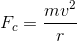The equation for magnetic force is: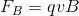Set the equations equal to each other, and solve for velocity.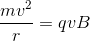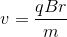The only answer choice that does not correspond to an increase in velocity is increasing the mass of the particles in the accelerator.